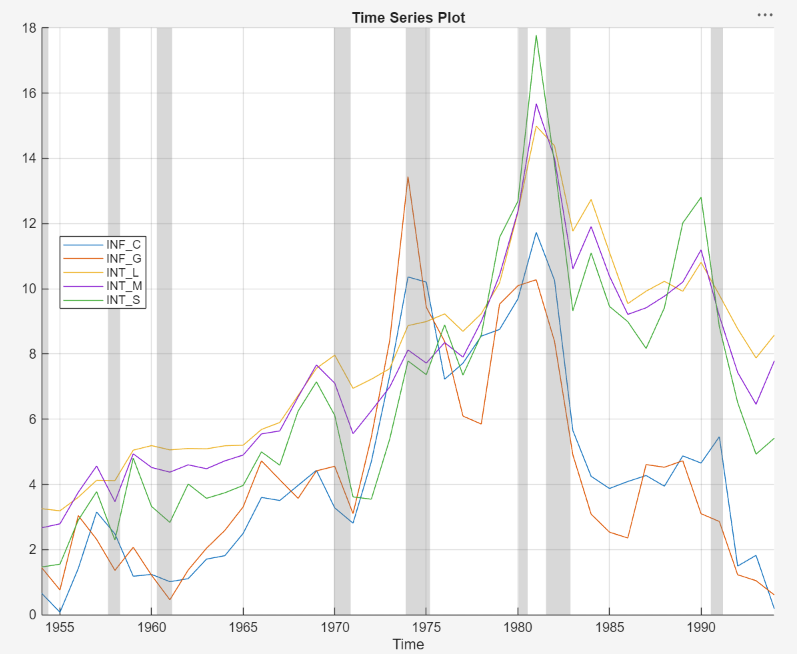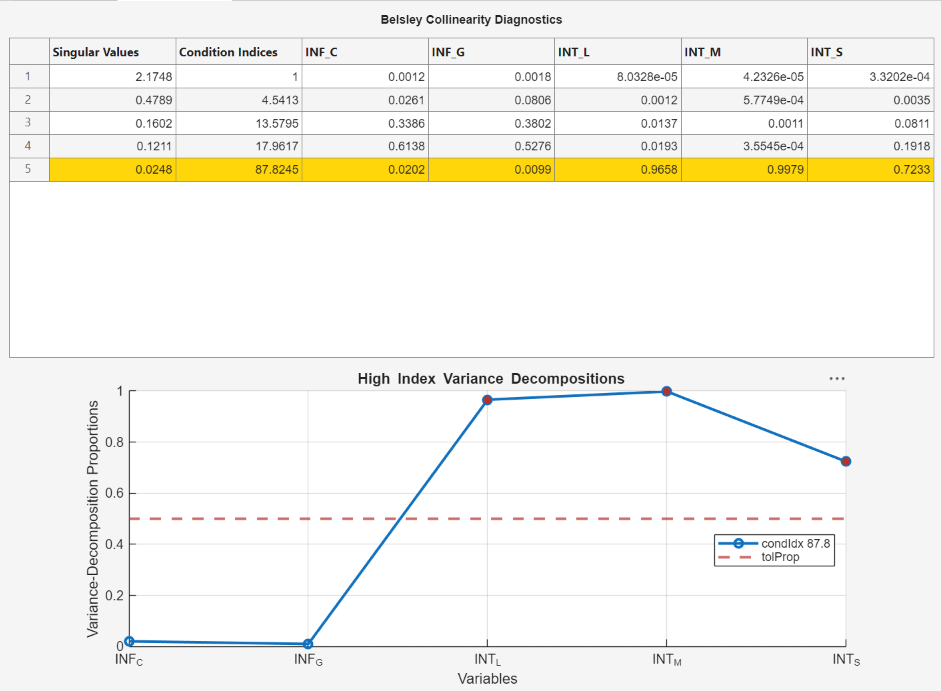Documentation

### This is machine translation

Mouseover text to see original. Click the button below to return to the English version of the page.

## Assess Collinearity Among Multiple Series Using Econometric Modeler App

This example shows how to assess the strengths and sources of collinearity among multiple series by using Belsley collinearity diagnostics in the Econometric Modeler app. The data set, stored in `Data_Canada`, contains annual Canadian inflation and interest rates from 1954 through 1994.

At the command line, load the `Data_Canada.mat` data set.

`load Data_Canada`

Convert the table `DataTable` to a timetable:

1. Clear the row names of `DataTable`.

2. Convert the sampling years to a `datetime` vector.

3. Convert the table to a timetable by associating the rows with the sampling times in `dates`.

```DataTable.Properties.RowNames = {}; dates = datetime(dates,12,31,'Format','yyyy'); DataTable = table2timetable(DataTable,'RowTimes',dates);```

At the command line, open the Econometric Modeler app.

`econometricModeler`

Alternatively, open the app from the apps gallery (see Econometric Modeler).

Import `DataTable` into the app:

1. On the Econometric Modeler tab, in the Import section, click.

2. In the Import Data dialog box, in the Import? column, select the check box for the `DataTable` variable.

3. Click .

The Canadian interest and inflation rate variables appear in the Data Browser, and a time series plot of all the series appears in the Time Series Plot(INF_C) figure window.Perform Belsley collinearity diagnostics on all series. On the Econometric Modeler tab, in the Tests section, click > Belsley Collinearity Diagnostics.

The Collinearity(INF_C) document appear with the following results:

• A table of singular values, corresponding condition indices, and corresponding variable variance-decomposition proportions

• A plot of the variable variance-decomposition proportions corresponding to the condition index that is above the threshold, and a horizontal line indicating the variance-decomposition thresholdThe interest rates have variance-decomposition proportions exceeding the default tolerance, 0.5, indicated by red markers in the plot. This result suggests that the interest rates exhibit multicollinearity. If you use the three interest rates as predictors in a linear regression model, then the predictor data matrix can be ill conditioned.